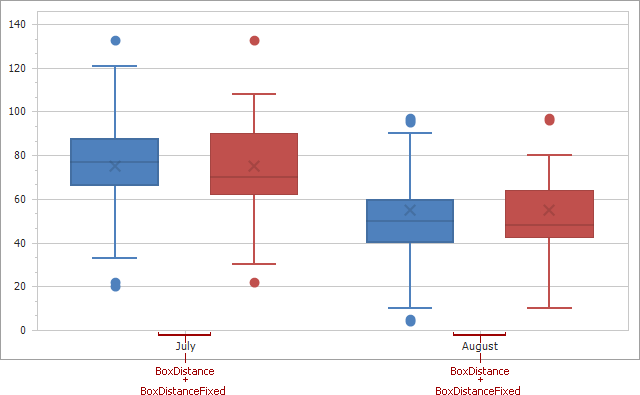# BoxPlotSeriesView.BoxDistance Property

Gets or sets the variable distance value (as a fraction of axis units) between two points of different Box Plot series shown at the same argument point.

Namespace: DevExpress.XtraCharts

Assembly: DevExpress.XtraCharts.v22.2.dll

NuGet Package: DevExpress.Charts

## Declaration

``````[XtraChartsLocalizableCategory(XtraChartsCategory.Layout)]
public double BoxDistance { get; set; }``````

## Property Value

Type Description
Double

A Double value that represents the distance between two neighboring points as a fraction of axis units, where an axis unit is the distance between two major values on the axis.

## Remarks

The BarDistance property specifies the distance between two points in a Box Plot series. This property’s value is given as a fraction, allowing the distance to be automatically resized when the size of the Chart is changed. For instance, if the BarDistance property is set to 0.5, then the distance between the two points will be always equal to half the range between the two major values on the axis.

To specify the distance between two bars in pixels, use the BoxPlotSeriesView.BoxDistanceFixed property. If both the BarDistance and BoxPlotSeriesView.BoxDistanceFixed properties are specified, their values are accumulated together as constant and variable distances.Note

The BarDistance property is synchronized in all Box Plot series in a ChartControl, and all of them always return the same value. So, changing the BarDistance property for any such series, affects the BarDistance property value in all other similar series.

For the same reason, when trying to set the BarDistance property before a Series is added to a chart’s collection, an ArgumentException is thrown.